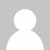In my previous post, I described a couple of options for creating a sound-wave icon graphic in XAML. While my final solution worked OK, I have since thought up an even simpler approach by using the XAML Path mini-language.

The reason I didn’t initially go for this option was that I didn’t want to calculate the coordinates of each point. However, we can draw the shape rotated by 45 degrees first, which allows us to put each point nicely on a grid, and then use a RotateTransform afterwards to put it back.

The XAML Path is a very useful tool to master if you prefer to work directly with XAML instead of using a design tool like Expression Blend. Here’s the first path, to create a quadrant shape:

``````<Path Fill="Orange" Data="M 0,0 h 1 a 1,1 90 0 1 -1,1 Z" />
``````

The M command starts us off by moving to the specified coordinates (0,0 in this case). A lower case h means a relative horizontal line – we move one unit to the right. Now the lower case a command means we are drawing an arc using relative coordinates. The first two arguments are the radius of the arc (1,1 = circle of radius 1), then comes the angle in degrees (90), then a flag indicating if this is a large arc – in our case, no (0), and then another flag indicating if we are going clockwise (1 = yes). Finally, we put the end coordinates (-1,1) which are relative to the starting point because we used a lower case a. This path is then closed with the Z command although this is not strictly necessary for us as we are not using a Stroke brush on this Path.

Now we can draw the other three sections, each of which consists of a horizontal line followed by an arc, then a vertical line, and finally another arc with a smaller radius. I don’t bother with the Z because I have reached my starting point again with the second arc.

``````<Path Fill="Orange" Data="M 2,0 h 1 a 3,3 90 0 1 -3,3 v -1 a 2,2 90 0 0 2,-2" />
<Path Fill="Orange" Data="M 4,0 h 1 a 5,5 90 0 1 -5,5 v -1 a 4,4 90 0 0 4,-4" />
<Path Fill="Orange" Data="M 6,0 h 1 a 7,7 90 0 1 -7,7 v -1 a 6,6 90 0 0 6,-6" />
``````

We end up with the right graphics but with the wrong orientation:A RotateTransform (plus a ScaleTransform) allows us to put this exactly how we want it:Here’s the complete XAML:

``````<Page
xmlns="http://schemas.microsoft.com/winfx/2006/xaml/presentation"
xmlns:x="http://schemas.microsoft.com/winfx/2006/xaml" Background="#E0E0E0">
<Grid Width="8" Height="8">

<Grid.RenderTransform>
<TransformGroup>
<ScaleTransform ScaleX="10" ScaleY="10" />
<RotateTransform Angle="-45" />
</TransformGroup>
</Grid.RenderTransform>

<Path Fill="Orange" Data="M 0,0 h 1 a 1,1 90 0 1 -1,1 Z" />
<Path Fill="Orange" Data="M 2,0 h 1 a 3,3 90 0 1 -3,3 v -1 a 2,2 90 0 0 2,-2" />
<Path Fill="Orange" Data="M 4,0 h 1 a 5,5 90 0 1 -5,5 v -1 a 4,4 90 0 0 4,-4" />
<Path Fill="Orange" Data="M 6,0 h 1 a 7,7 90 0 1 -7,7 v -1 a 6,6 90 0 0 6,-6" />
</Grid>
</Page>
``````